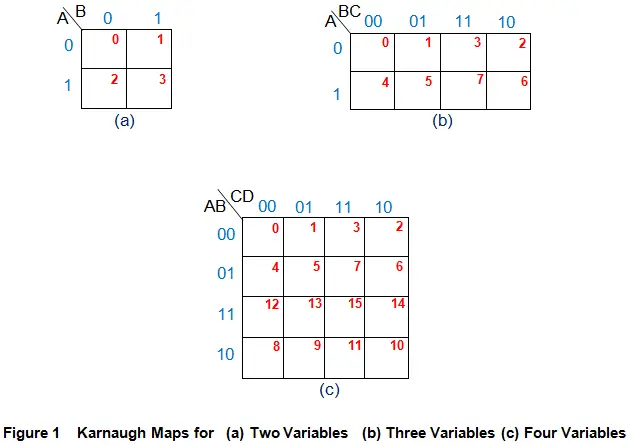# K Map or Karnaugh Map

Simplification of Boolean expressions is an important step while designing any digital system. Karnaugh Maps or K-maps is one among such simplification technique, introduced by Maurice Karnaugh in 1953, which is graphical in nature. This method of minimizing the logical expressions is most suitable when the number of variables involved is less than or equal to four.

This is because, a K-map employs the use of two-dimensional tables to simplify the expressions, whose size increases at a very high rate with the increase in the number of variables. This fact is further established by Figure 1 which shows the K-maps for two, three and four variables in order.From the figure, it is evident that the number of cells in the K-map is a function of number of inputs. In general, if there are n inputs, then the corresponding K-map has to be of 2n cells. For example, if the number of input variables is 2, then we have to consider a K-map with 4 (=22) cells, while if there are 3 input variables, then we require a 8 (=23) cell K-map, and similarly for 4 inputs one gets 16 (=24) cell K-map and so on.

## Structure of K-map

All the K-maps irrespective of their size, are seen to possess a generalized structure (Figure 1). Every K-map has a set of input variables represented at its top left-corner (black alphabets). These are the input variables which are involved in the logical expression which needs to be simplified. The value(s) of these variable(s) is/are shown in binary along their respective sides (combination of zeros and ones shown in blue).

Here, it is seen that the binary patterns of any two adjacent cells differ by just a single bit. This kind of encoding scheme is referred to as gray code and is employed in order to ease the process of grouping which inturn minimizes the logical expression.

Further these binary sequences are seen to assign a definite input bit pattern for each K-map cell, whose decimal equivalent is shown in red numbers inside each of them. For example, third cell of the first row in Figure 1b corresponds to the input bit pattern ABC = 011 which is represented by its decimal equivalent 3.

K-map simplification procedure is initiated by entering the values of the output variable (either for sum-of-products, SOP or for product-of-sums, POS) in appropriate K-map cells. Then one has to group the maximum number of ‘ones’ (incase of SOP) or ‘zeros’ (incase of POS). T

hese groups should necessarily be in powers of 2 and should be carried on in descending order only. For instance, if there are 8 cells in the K-map, then, first, try grouping for 8 (=23), then for 4 (=22), next for 2 (=21) and lastly consider the isolated terms. After this, each group is expressed interms of the combination of input variables which correspond to the common binary values along the associated rows and columns. Finally, these are used to express the output of the logical expression.

### Advantages of Karnaugh Map

Advantages of K-map are showing below

1. K-map simplification does not demand for the knowledge of Boolean algebraic theorems.
2. Usually it requires less number of steps when compared to algebraic minimization technique.

### Disadvantages of Karnaugh Map

Disadvantages of K-map are showing below

1. Complexity of K-map simplification process increases with the increase in the number of variables
2. The minimum expression obtained might not be unique

Want To Learn Faster? 🎓
Get electrical articles delivered to your inbox every week.
No credit card required—it’s 100% free.